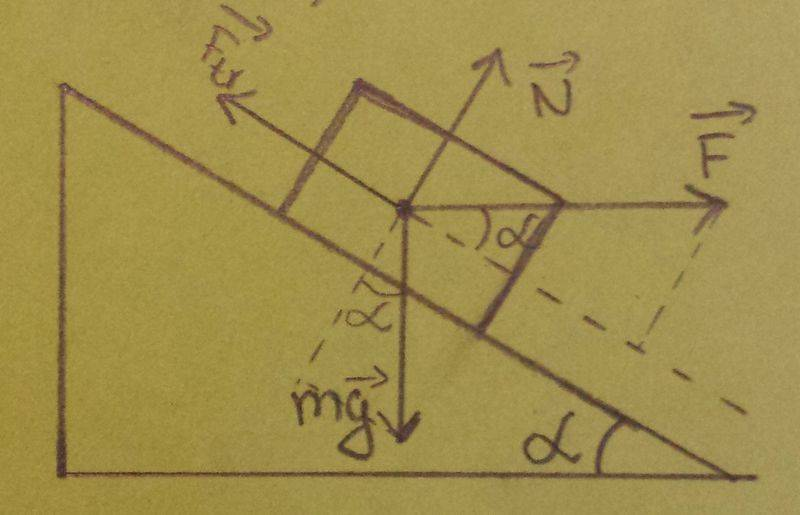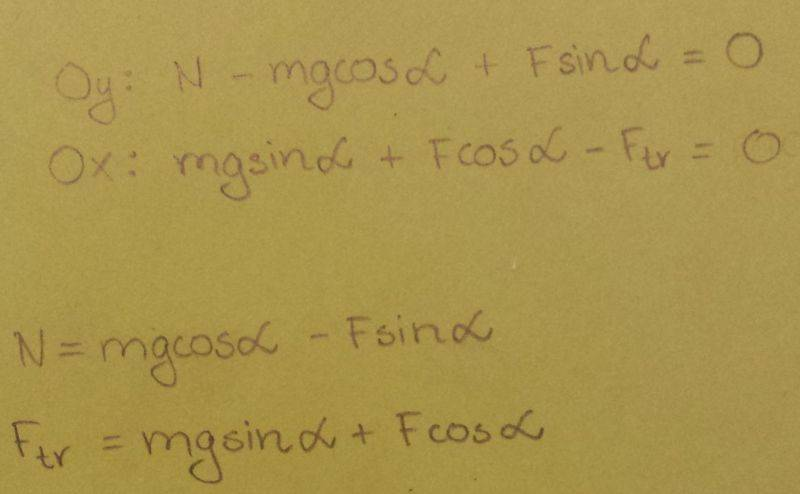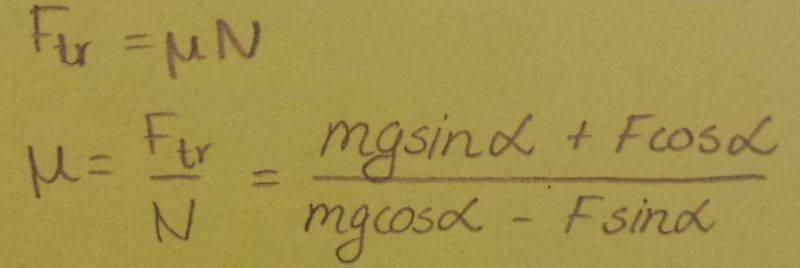# Forces and Laws of Motion

## Homework Statement

A 225 kg crate rests on a surface that is inclined above the horizontal at an angle of 20o.
A horizontal force (F = 535 N parallel to the ground, not the incline) is required to start the crate moving down the incline. What is the coefficient of friction between the crate and the incline?

P.S. Ftr is a frictional force.## Homework Equations

Newton's laws of motion.

## The Attempt at a SolutionIt doesn't work.
The answer must be 0.665 .[/B]